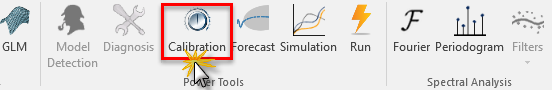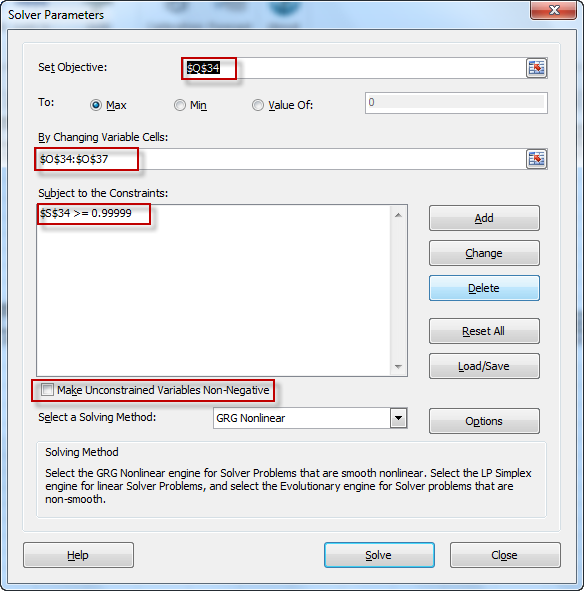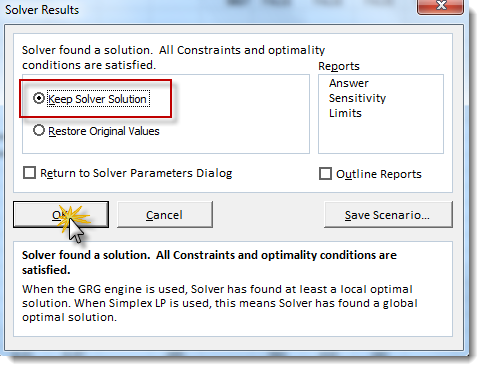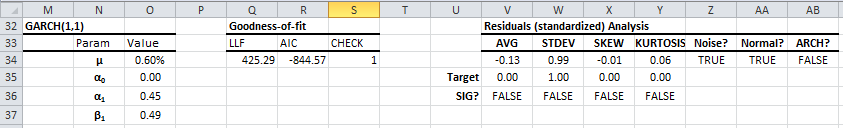# Module 6 - Model Calibration

In module five (5), we presented a few steps to specify the time series model, along with goodness-of-fit and residuals diagnosis tables.

In this module, we will continue along this path and find the optimal values for the model’s parameters – a process referred to as “calibration”. Once calibrated, you can examine the residuals for the model’s assumptions and compare this model with other models.

NumXL supports numerous time series models, but fortunately, the calibration process using NumXL is the same for all models.

In a nutshell, calibration is an optimization problem where we search for a set of parameter values that maximize the value of a utility function (i.e. log-likelihood function) while complying with one constraint: the stability of the model.

What do we mean by model stability? For an ARMA model, the underlying process has a finite unconditional (long-run) mean and variance (i.e. the roots of the characteristics equation are outside the unit circle). For GARCH and GARCH-variant models, in addition to the constraint earlier, the variance model must guarantee positive values for a conditional variance.

Fortunately, NumXL lumps together model-specific constraints with a function (e.g. ARMA_CHECK, GARCH_CHECK, etc.). The function returns one(1) for a stable model, otherwise zero(0).

In the Goodness-of-fit table, the right-most formula is actually a stability check.The first two tables contain all inputs we need to carry on the calibration process, so let’s process.

1. Select the top cell in the model table (i.e. M32 in the figure above).
2. Locate and click on the calibration icon in the NumXL toolbar.3. The Microsoft Excel solver pops up in your worksheet.4. Notice that all fields in the solver are already pre-set with your model
5. Click on the Solver button

The solver begins its search for a set of parameter values that maximize the objective (i.e. log-likelihood function) while keeping the model valid/stable.

Using the GRG method, the solver does not guarantee global maxima and we may end up with a locally optimal solution. This is sufficient for the majority of potential cases.Once we accept the solver solution, the new set of parameter values are copied to your worksheet.

Note: If the calculation options are set to manual, you need to force recalculate to update all formulas that reference the model’s parameter cells.

The calibrated model is shown below:Note that the LLF function changed from 412 to 425 value with the new optimal values, yet the model is valid/stable (S34 = 1).

## What’s next?

The calibrated parameters’ values improved the overall fit of the model with input data, but is this the right model? We need to examine the assumption(s) of GARCH and whether they are met with this model or not. This will be the focus of our next module